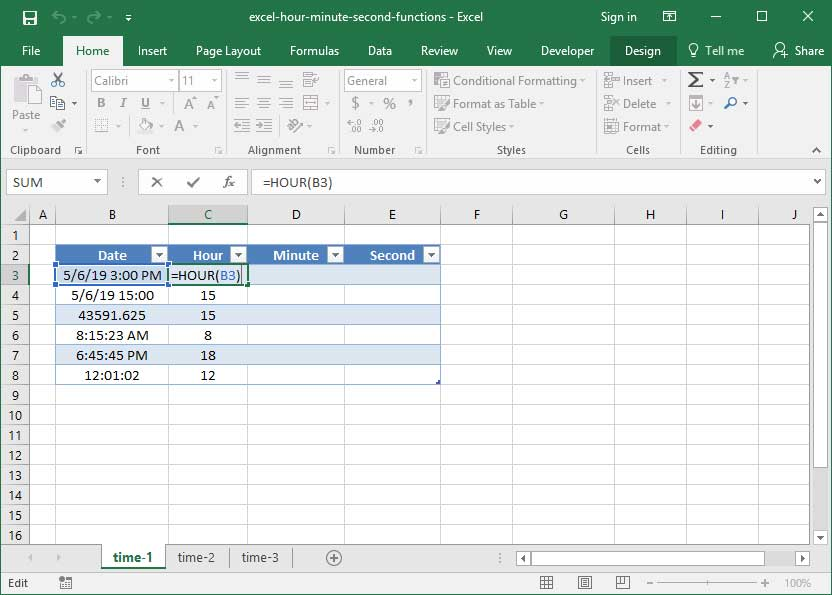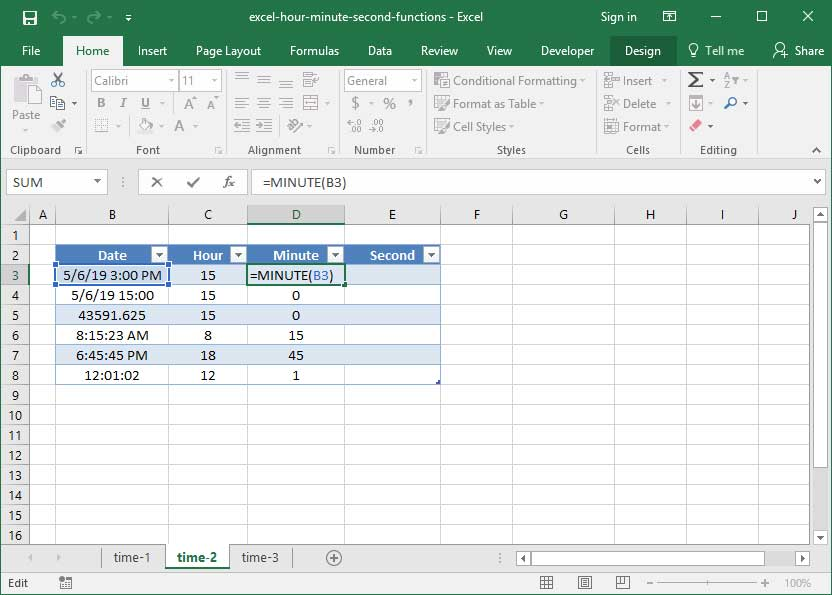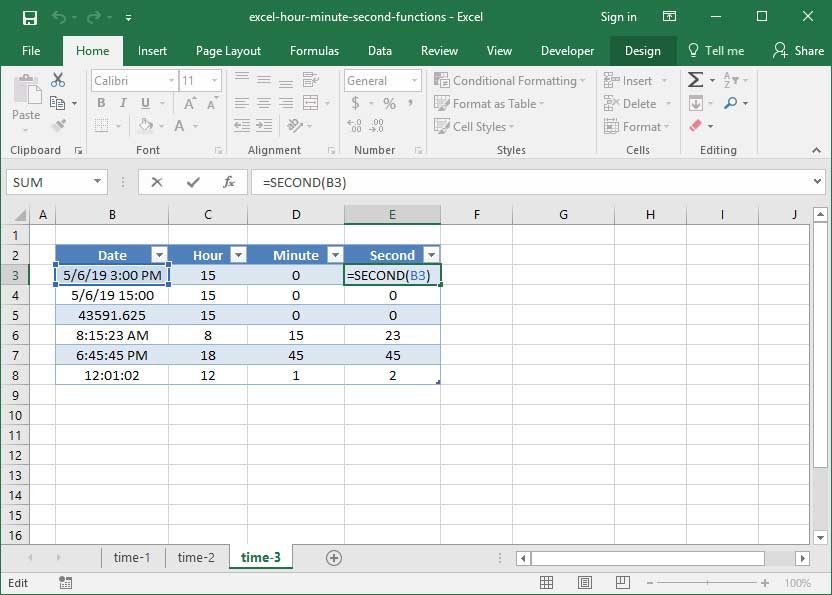# Excel's HOUR, MINUTE, and SECOND functions

Excel's HOUR, MINUTE, and SECOND functions work much the same as YEAR, MONTH, and DAY — they take a date or time as an input (formatted as a serialized date and timestamp) and convert it into individual hour, minute, and second readings.

Before reading through this tutorial, it's critical that you familizarize yourself with Excel's time formatting and serialization techniques. If you need to do so, head on over to our TIME function overview first. It can also be helpful to read our YEAR, MONTH, and DAY tutorial for additional context.

## The HOUR function

Like YEAR, MONTH, and DAY, HOUR takes a time-formatted or serialized input and outputs the hour number in 24-hour time format. Recall from our TIME function overview that Excel's time format comes in the format HH:MM:SS, where HH represents hours, MM represents minutes, and SS represents seconds. You can either add an AM / PM designation to the end, or specify times in 24-hour format.

The syntax of the HOUR function is:

`=HOUR(serial_number)`

HOUR can be used on either a time-formatted string, or on a serial number, like so (note that the serialized version of 5/6/2019 3:00:00 PM is 43591.625):

`=HOUR("5/6/2019 3:00:00 PM")Output: 15`
`=HOUR(43591.625)Output: 15`

The above formula outputs the number 15, because 3:00:00 PM is the 15th hour of the day according to the 24-hour time clock. Remember that each hour is approximately equal to 0.41666..., since 1/24 is equal to 0.41666....

Additionally, note that we can use HOUR on a time-formatted object with no date, like so:

`=HOUR("11:30 AM")Output: 11`
`=HOUR("0.491666")Output: 11`

Below, take a look at how we use HOUR on a number of time formats:`=HOUR(B3)Output: 15`

Per the above, our HOUR function works on a variety of time formats, including 5/6/19 3:00 PM, 43591.635, and 12:01:02.

## The MINUTE and SECOND functions

The MINUTE and SECOND functions work exactly the same as HOUR above: they take in a time-formatted or serialized input and output minute and second numbers. Their syntax is similar to HOUR:

`=MINUTE(serial_number)`
`=SECOND(serial_number)`

Let's take a look at a couple of sample formulas using these functions (note that the serialized version of 5/6/2019 8:15:00 AM is 43591.34375, and the serialized version of 5/6/2019 8:15:30 AM is 43591.3441):

`=MINUTE("5/6/2019 8:15:00 AM")Output: 15`
`=MINUTE(43591.34375)Output: 15`
`=SECOND("5/6/2019 8:15:00 AM")Output: 0`
`=SECOND(43591.34375)Output: 0`
`=SECOND("5/6/2019 8:15:30 AM")Output: 30`
`=SECOND(43591.3441)Output: 30`

And, finally, we'll apply SECOND and MINUTE to our list of time-formatted cells above:`=MINUTE(B3)Output: 0``=SECOND(B3)Output: 0`

Now you know the basics of Excel's HOUR, MINUTE, and SECOND functions. Please let us know if you have any questions or thoughts in the Comments section below!

## Save an hour of work a day with these 5 advanced Excel tricks

Work smarter, not harder. Sign up for our 5-day mini-course to receive must-learn lessons on getting Excel to do your work for you.

• How to create beautiful table formatting instantly...
• Why to rethink the way you do VLOOKUPs...
• Plus, we'll reveal why you shouldn't use PivotTables and what to use instead...